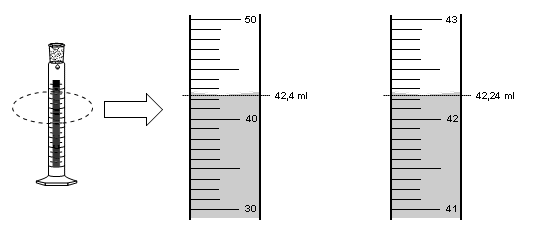The results obtained in a measurement are not exact. Every measurement implies an estimate. For example, suppose we need to measure an object with a ruler graduated in millimeters. When measuring we obtain a result between 38 and 39 millimeters, we estimate that the object measures 38.5 millimeters. This result has an exact part 38 and a part that is estimated (approximate) which is the last digit 5. The number 38.5 mm contains three significant figures. The last digit is doubtful, but is considered a significant figure. When giving the result of a measurement we include an approximate digit, but only one.

Next we will use a measuring cylinder to measure volumes of liquids. To the right of the specimen the calibration lines are enlarged. On the right scale we move from 10 ml to 10 ml. The left scale is graduated so that it varies from milliliter to milliliter.We are going to make a measurement using both calibration scales. On the left scale, the liquid level is above 42 ml, since there are no calibration lines between 42 ml and 43 ml, we estimate the last digit and give it a value of 4. The measurement value is 42.4 ml.

In the second calibration scale, the liquid level exceeds the 42.2 line. Since there is no scale between 42.2 and 42.3, we estimate the fourth digit of our measurement at 4. The value of the measurement is 42.24 ml.

On the left scale, the measurement result has three significant figures, the last one being an estimate. The right scale gives results to four significant figures, the last figure being an estimate.